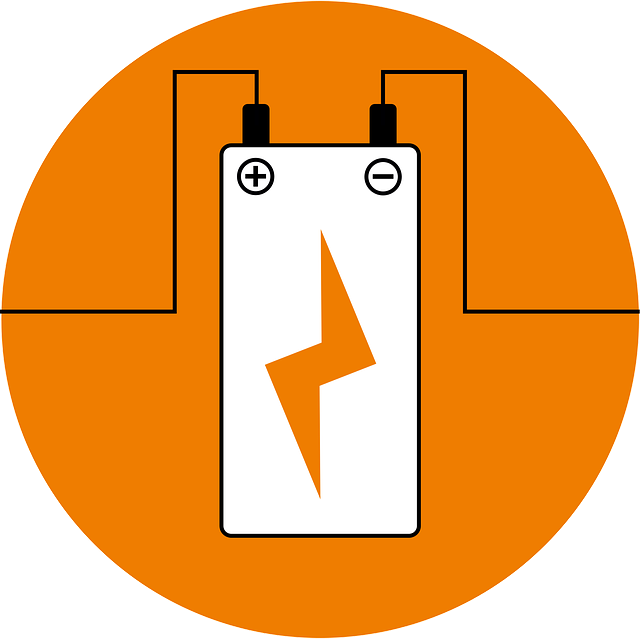# Current Electricity for O Level Physics – What’s testedIn this post, let’s look at what’s tested in detail for current electricity for O Level physics.

I go through the points in detail in my video here.

This is a chapter with mainly calculations, hence, knowing all the formulae is essential. You can find a list of formulae for O Level Physics here. With new terms introduced e.g. current, potential difference, etc., comes the requirement to know how to define them. Find a list of definitions for O Level Physics here.

Below is a checklist of what you need to know for O Level Physics on the topic current electricity.

## Checklist for Current Electricity for O Level Physics

✓ define current

✓ differences between conventional current and electron flow

✓ formula: Q = It, where Q = charge, I = current, t = time

✓ define electromotive force (e.m.f) and potential difference (p.d)

✓ formula: V = E/Q, where V is e.m.f or p.d., E. = work done or energy dissipated, Q = charge

✓ Ohms law

✓ Resistance, and how resistance is affected by length, cross sectional area, and material

✓ formula: V = IR, where V is p.d., I is current and R is resistance

✓ finding effective resistance for resistors connected in series and parallel

✓ describe experiment to determine resistance

✓ effect of temperature on resistance

✓ sketch and interpret I/V graphs for ohmic conductor, lamp and semiconductor diodes

Watch the video below to learn current electricity in detail.

error: Content is protected !!